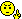# Math Is Fun Forum

Discussion about math, puzzles, games and fun.   Useful symbols: ÷ × ½ √ ∞ ≠ ≤ ≥ ≈ ⇒ ± ∈ Δ θ ∴ ∑ ∫  π  -¹ ² ³ °

You are not logged in.

## #1 2023-11-21 03:46:28

sologuitar
Member
Registered: 2022-09-19
Posts: 467

### Standard Form a + bi

Write each expression in the standard form a + bi.

A. i^(23)

I say i^(23) can be expressed as (i^5)(i^4)(i^3).

i^5 = i

i^4 = 1

i^3 = -i

(i)(1)(-i) = (i)(-i) = -(i^2) = i(-1) = -I

You say?

B. i^(-15)

I think the first step is to remove the negative power.

So, i^(-15) = 1/(i^15)

i^(15) = (i^5)^3

i^5 = i

I now have 1/(i)^3 = 1/(i^3) = 1/-i

What did I do wrong with B?

Last edited by sologuitar (2023-11-21 03:47:22)

Offline

## #2 2023-11-21 06:17:44

BobRegistered: 2010-06-20
Posts: 9,863

### Re: Standard Form a + bi

I say i^(23) can be expressed as (i^5)(i^4)(i^3).add the powers  (i^5)(i^4)(i^3) = i^(5+4+3)  ≠  i^23

i^4 = (i x i) x (i x i) = -1 x -1 = 1

23 = 4 + 4 + 4 + 4 + 4 + 3

so i^23 = i^3

The second one is ok up to i^5 = i

i^3 = (i x i) x i = -i

so you have 1/(-i)  If you times top and bottom by -i you can simplify this.

Bob

Children are not defined by school ...........The Fonz
You cannot teach a man anything;  you can only help him find it within himself..........Galileo Galilei
Sometimes I deliberately make mistakes, just to test you!  …………….BobOffline

## #3 2023-11-21 07:11:21

amnkb
Member
Registered: 2023-09-19
Posts: 175

### Re: Standard Form a + bi

sologuitar wrote:

Write each expression in the standard form a + bi.

A. i^(23)

sologuitar wrote:

B. i^(-15)

Offline

## #4 2023-11-22 10:32:59

sologuitar
Member
Registered: 2022-09-19
Posts: 467

### Re: Standard Form a + bi

Bob wrote:

I say i^(23) can be expressed as (i^5)(i^4)(i^3).add the powers  (i^5)(i^4)(i^3) = i^(5+4+3)  ≠  i^23

i^4 = (i x i) x (i x i) = -1 x -1 = 1

23 = 4 + 4 + 4 + 4 + 4 + 3

so i^23 = i^3

The second one is ok up to i^5 = i

i^3 = (i x i) x i = -i

so you have 1/(-i)  If you times top and bottom by -i you can simplify this.

Bob

I see now what I did wrong.

Offline

## #5 2023-11-22 10:34:29

sologuitar
Member
Registered: 2022-09-19
Posts: 467

### Re: Standard Form a + bi

amnkb wrote:
sologuitar wrote:

Write each expression in the standard form a + bi.

A. i^(23)

sologuitar wrote:

B. i^(-15)

Nicely-done but try to explain what you're doing along the way.

Offline